# Outline

 TensorRT TensorRT 的優化方式 TensorRT vs Tensorflow Lite TensorRT建構流程 ONNX (Open Neural Network Exchange) Jetson Nano安裝TensorRT. PyTorch 匯出 ONNX. 透過ONNX RUNTIME運行ONNX Model 使用TensorRT運行ONNX PyTorch使用TensorRT最簡單的方式 YOLOv5使用TensorRT引擎方式 結語

# TensorRT

TensorRT是由 NVIDIA 所推出的深度學習加速引擎 ( 以下簡稱trt )，主要的目的是用在加速深度學習的 Inference，按照官方提出TensorRT比CPU執行快40倍的意思，就像是YOLOv5針對一張圖片進行推論用CPU的話大概是1秒，如果用上TensorRT的話可能就只要0.025秒而已，這種加速是非常明顯的！而這種加速大部分都是運用的邊緣裝置上 ( Edge Device )，由於邊緣裝置上的效能沒有一般電腦來的好，所以往往需要這種軟體加速。

#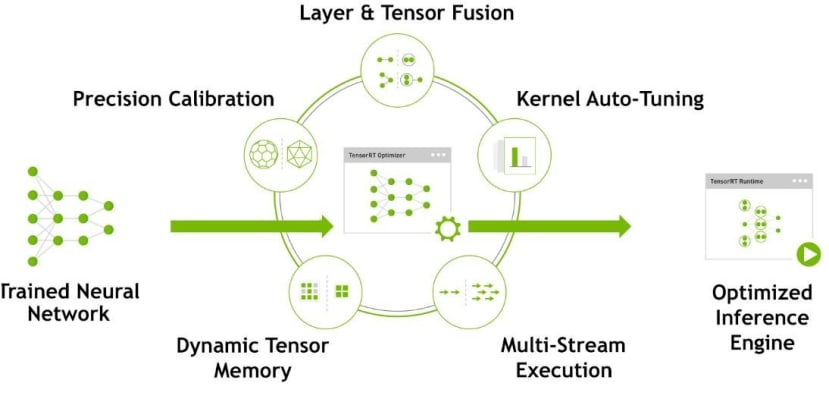TensorRT主要的優化過程有這些： Precision Calibration、Layer & Tensor Fusion、Kernel Auto-Tuning、Dynamic Tensor Memory、Multi-Stream Execution，今天會介紹前兩種，後面三種比較底層資訊也比較少所以就先不介紹了。

#### 對模型進行重構與優化（Layer & Tensor Fusion）

1.第一步先刪除沒有使用到的輸出層

2.垂直優化：由於CUDA會在每一層輸入的時候啟動，而這個垂直優化就是透過將常使用的層作合併，像圖中將Conv、bias、relu合併為CBR，減少CUDA啟動關閉的次數來達到時間上的縮減。3.水平優化：理論同上，如果同層其他分支也在運作一樣的架構則直接合併，要注意的地方是合併成同一層之後輸出必須要做分割，在輸出到不同層去。# TensorRT建構流程

1. ONNX parser：將模型轉換成ONNX的模型格式。
2. Builder：將模型導入TensorRT並且建構TensorRT的引擎。
3. Engine：引擎會接收輸入值並且進行Inference跟輸出。
4. Logger：負責記錄用的，會接收各種引擎在Inference時的訊息。

# ONNX (Open Neural Network Exchange)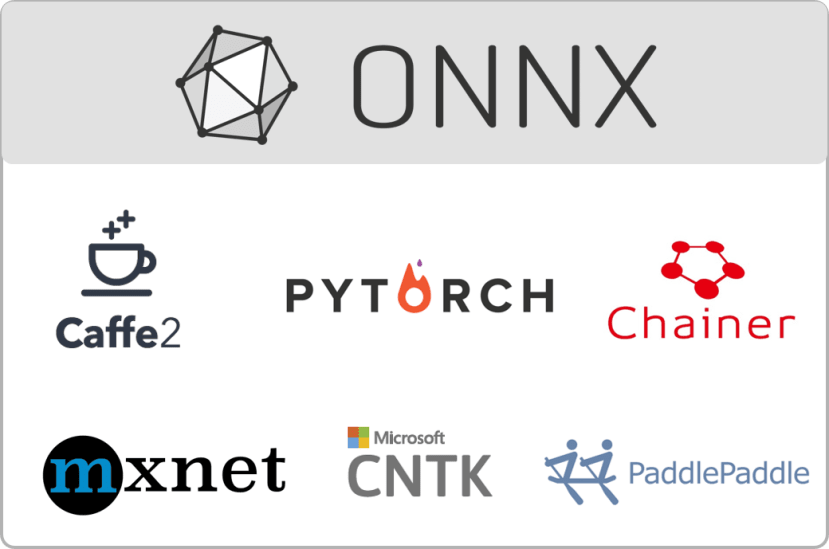# Jetson Nano安裝TensorRT

#### 1.  官方作法https://docs.nvidia.com/deeplearning/tensorrt/install-guide/index.html#installing-tar

#### 2.  我的映像檔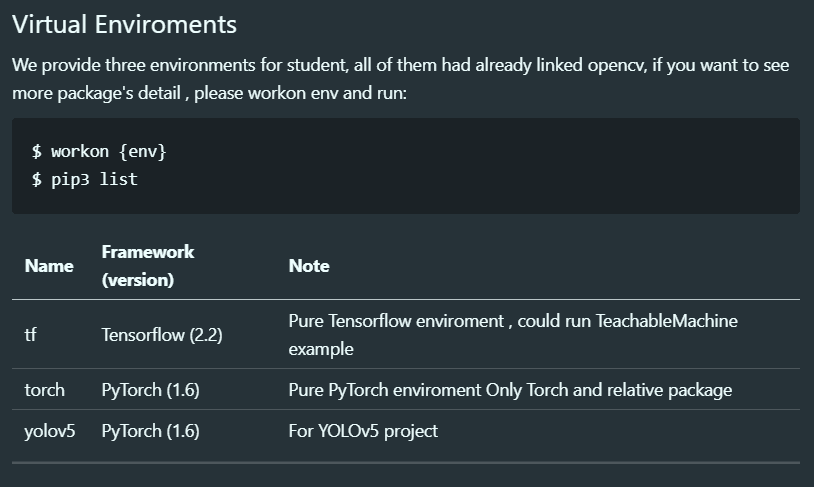#### 將TensorRT連結到virtualenv

``````\$ vim ~/.bashrc
export CUDA_HOME=/usr/local/cuda
export PATH=\$CUDA_HOME/bin:\$PATH
export LD_LIBARY_PATH=\$CUDA_HOME/lib64:\$LD_LIBRARY_PATH
export PYTHONPATH=/usr/lib/python3.6/dist-packages:\$PYTHONPATH
``````

# PyTorch 匯出 ONNX

#### Torch.onnx.export

``torch.onnx.export(model, args, f, export_params=True, verbose=False, training=False, input_names=None, output_names=None, aten=False, export_raw_ir=False, operator_export_type=None, opset_version=None, _retain_param_name=True, do_constant_folding=False, example_outputs=None, strip_doc_string=True, dynamic_axes=None, keep_initializers_as_inputs=None)``

#### 使用方法

``````import torch
import torchvision

dummy_input = torch.randn(1, 3, 224, 224, device='cuda')
model = torchvision.models.alexnet(pretrained=True).cuda()

input_names = [ "actual_input_1" ] + [ "learned_%d" % i for i in range(16) ]
output_names = [ "output1" ]

torch.onnx.export(model, dummy_input, "alexnet.onnx", verbose=True, input_names=input_names, output_names=output_names)
``````The resulting alexnet.onnx is a binary protobuf file which contains both the network structure and parameters of the model you exported (in this case, AlexNet). The keyword argument verbose=True causes the exporter to print out a human-readable representation of the network:

#### 檢查ONNX模型

``\$ pip install onnx``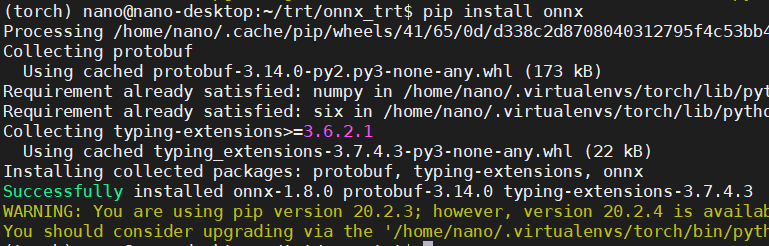``````import onnx

# Check that the IR is well formed
print(onnx.checker.check_model(model))

print('-'*50)

# Print a human readable representation of the graph
print('Model :\n\n{}'.format(onnx.helper.printable_graph(model.graph)))
``````#### 圖形化顯示ONNX模型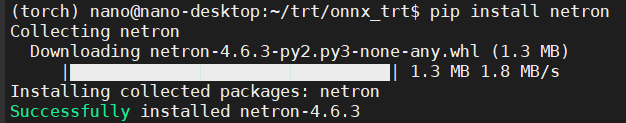``\$ netron [FILE]``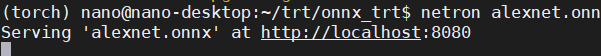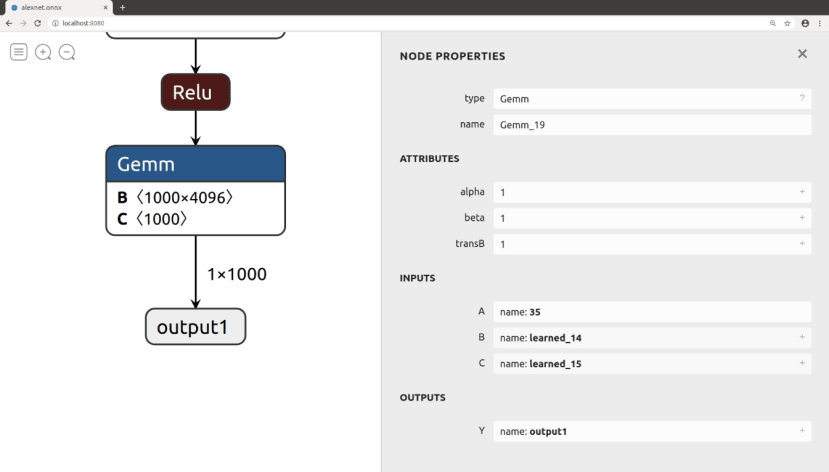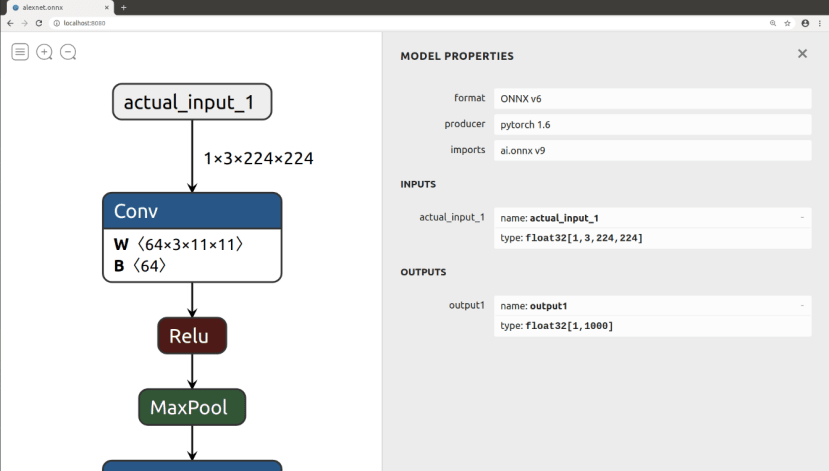# 透過ONNX RUNTIME運行ONNX Model

``\$ pip install onnxruntime``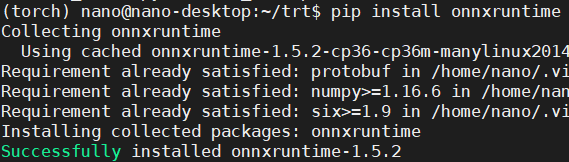``````import onnxruntime as ort
ort_session = ort.InferenceSession('alexnet.onnx')
outputs = ort_session.run(None, {'actual_input_1': np.random.randn(10, 3, 224, 224).astype(np.float32)})
print(outputs)
``````

#### ORT 運行 AlexNet

``````import time

def print_div(text, custom='', end='\n\n'):
print('-'*50, '\n')
print(f"{text}{custom}", end=end)

class timer():

def __init__(self, text):
print_div(text, '...', end=' ')
self.t_start = time.time()

def end(self):
t_cost = time.time() - self.t_start
print('\033[35m', end='')
print('Done ({:.5f}s)'.format(t_cost))
print('\033[0m')

class logger():

def __init__(self, text):
print_div(text)
``````

``````import onnxruntime as ort
import time
from PIL import Image
import numpy as np
from torchvision import transforms as T

# Custom
from log import timer, logger
``````

``````trans = T.Compose([T.Resize(256), T.CenterCrop(224), T.ToTensor()])
img = Image.open('../test_photo.jpg')
img_tensor = trans(img).unsqueeze(0)
img_np = np.array(img_tensor)
logger('Image : {} >>> {}'.format(np.shape(img) , np.shape(img_tensor)))
``````

``````# ONNX Run Time
ort_session = ort.InferenceSession('alexnet.onnx')

# run( out_feed, in_feed, opt )
input_name = ort_session.get_inputs().name

infer_onnx = timer('Run Infer')
outputs = ort_session.run(None, {input_name: img_np})
infer_onnx.end()
``````

``````# Get Labels
f = open('../imagenet_classes.txt')
t = [ i.replace('\n','') for i in f.readlines()]
logger("Result : {}".format(t[np.argmax(outputs)]))
``````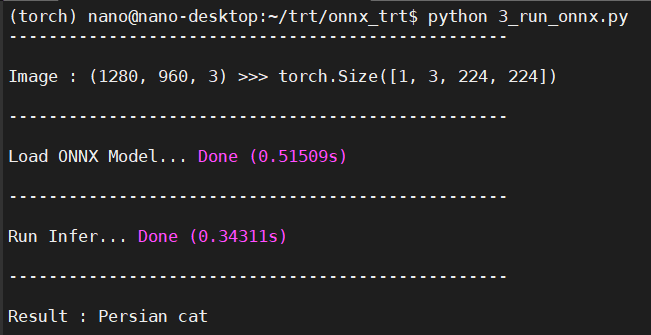#### ORT運行.onnx與PyTorch運行.pth速度差異#### 參考文章

What is the difference between tflite and tensorRT?

TensorRT(1)-介绍-使用-安装

TensorRT-Optimization-Principle

Speeding up Deep Learning Inference Using TensorFlow, ONNX, and TensorRT

### 下载

CAVEDU Education is devoted into robotics education and maker movement since 2008, and is intensively active in teaching fundamental knowledge and skills. We had published many books for readers in all ages, topics including Deep Learning, edge computing, App Inventor, IoT and robotics. Please check CAVEDU's website for more information: http://www.cavedu.com, http://www.appinventor.tw